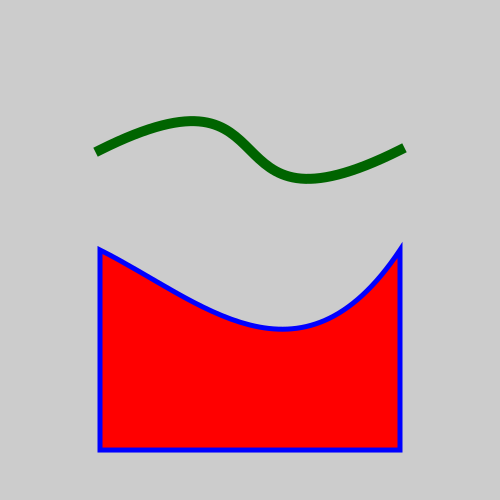# Bezier

Martin McBride, 2020-10-25
Tags geometry bezier curve
Categories generativepy generative artThe Bezier class draws a bezier curve.

A bezier curve is based on 4 control points, a, b, c and d. The curve starts at point a and ends at point d. The points b and c are called the handles, and they control the shape of the curve.

Here is a bezier curves tutorial that covers this in a lot more detail.

## Bezier class methods

The Bezier class inherits add, fill, stroke, fill_stroke, path, clip and other methods from Shape.

• of_abcd
• of_bcd

### of_abcd

Creates a line based on the 4 control points.

of_abcd(a, b, c, d)

Parameter Type Description
a (number, number) A tuple of two numbers, giving the (x, y) position of the start of the curve.
b (number, number) A tuple of two numbers, giving the (x, y) position of the first handle.
c (number, number) A tuple of two numbers, giving the (x, y) position of the second handle.
d (number, number) A tuple of two numbers, giving the (x, y) position of the end of the curve.

### of_bcd

Creates a line based on the handles and end point only.

of_bcd(b, c, d)

Parameter Type Description
b (number, number) A tuple of two numbers, giving the (x, y) position of the first handle.
c (number, number) A tuple of two numbers, giving the (x, y) position of the second handle.
d (number, number) A tuple of two numbers, giving the (x, y) position of the end of the curve.

This method creates a curve by specifying the handles and end point only. The start point is set to a value of (0, 0).

This is mainly intended for use in composite paths, where lines are joined one after the other. Each line takes its start point as the previous lines end point. See the composite paths tutorial.

## Example

Here is some example code that draws a curve using the class. The full code can be found on github.

from generativepy.drawing import make_image, setup
from generativepy.color import Color
from generativepy.geometry import Bezier, Polygon

'''
Create bezier curve using the geometry module.
'''

def draw(ctx, width, height, frame_no, frame_count):
setup(ctx, width, height, width=5, background=Color(0.8))

# Bezier objects can only be stroked as they do not contain an area.
Bezier(ctx).of_abcd((1, 1.5), (3, 0.5), (2, 2.5), (4, 1.5)).stroke(Color('darkgreen'), 0.1)

# Create a polygon with a bezier side
Polygon(ctx).of_points([(1, 4.5), (1, 2.5), (2, 3, 3, 4, 4, 2.5), (4, 4.5)]).fill(Color('red')).stroke(Color('blue'), 0.05)

make_image("/tmp/geometry-bezier.png", draw, 500, 500)


Here is the image it creates:The top curve is created using the Bezier class.

The bottom shape is created using the Polygon class described here.

If you found this article useful, you might be interested in the book Computer Graphics in Python or other books by the same author.

#### Popular tags

2d arrays abstract data type alignment and angle animation arange arc array arrays behavioural pattern bezier curve built-in function callable object chain circle classes clipping close closure cmyk colour combinations comparison operator comprehension context context manager conversion count creational pattern data science data types design pattern device space dictionary drawing duck typing efficiency ellipse else encryption enumerate fill filter font font style for loop function function composition function plot functools game development generativepy tutorial generator geometry gif global variable gradient greyscale higher order function hsl html image image processing imagesurface immutable object in operator index inner function input installing iter iterable iterator itertools join l system lambda function len line linear gradient linspace list list comprehension logical operator lru_cache magic method mandelbrot mandelbrot set map matplotlib monad mutability named parameter numeric python numpy object open operator optimisation optional parameter or pandas partial application path pattern permutations polygon positional parameter print pure function python standard library radial gradient range recipes rectangle recursion reduce repeat rgb rotation roundrect scaling scipy sector segment sequence setup shape singleton slice slicing sound spirograph sprite square str stream string stroke structural pattern subpath symmetric encryption template text text metrics tinkerbell fractal transform translation transparency triangle truthy value tuple turtle unpacking user space vectorisation webserver website while loop zip